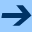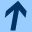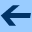Covariant embeddings of the linear functional equation with respect to an iteration group in the ring of complex formal power series

## Covariant embeddings of the linear functional equation with respect to an iteration group in the ring of complex formal power series

Jointly written with LUDWIG REICH.

Grazer Mathematische Berichte 350, 96 - 121, 2006.

Abstract: Let a(x), b(x), p(x) be formal power series in the indeterminate x over ℂ such that orda(x)=0, ordp(x)=1 and p(x) is embeddable into an iteration group (π(s,x))s∈ ℂ in C[[x]]. By a covariant embedding of the linear functional equation

(L) φ(p(x))=a(x)φ(x)+b (x),
(for the unknown series φ(x)∈ C[[x]]) with respect to (π(s,x))s∈ ℂ we understand families (α(s,x))s∈ ℂ and (β(s,x))s∈ ℂ of formal power series which satisfy a system of cocycle equations and boundary conditions such that
(Ls) φ(π(s,x))=α(s,x)φ(x)+β(s,x),        s∈ ℂ,
holds true for all solutions φ of (L). In this paper we present a complete solution of this problem and we demonstrate how earlier results concerning covariant embeddings with respect to analytic iteration groups can be derived from these more general results.
harald.fripertinger "at" uni-graz.at, October 10, 2019